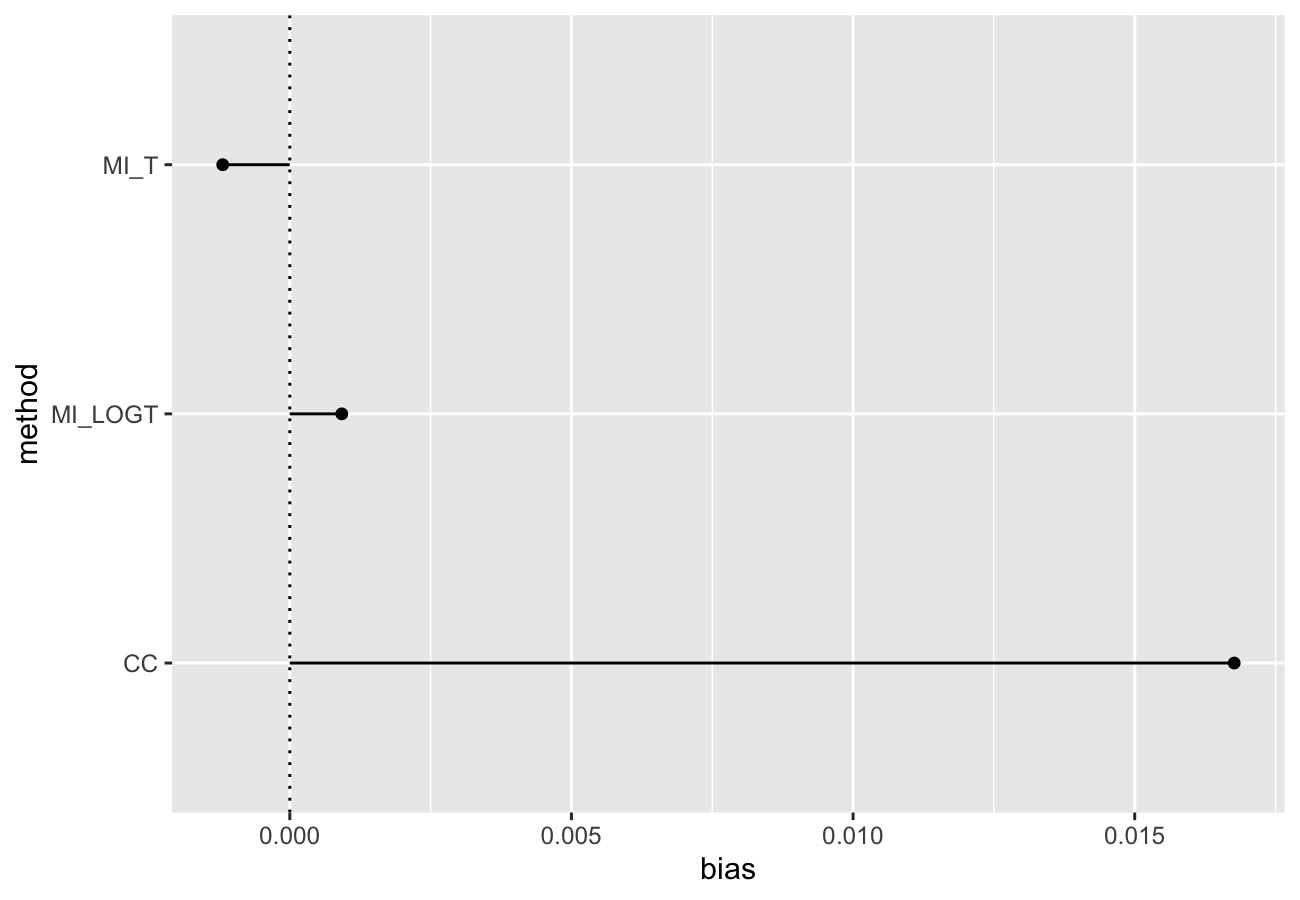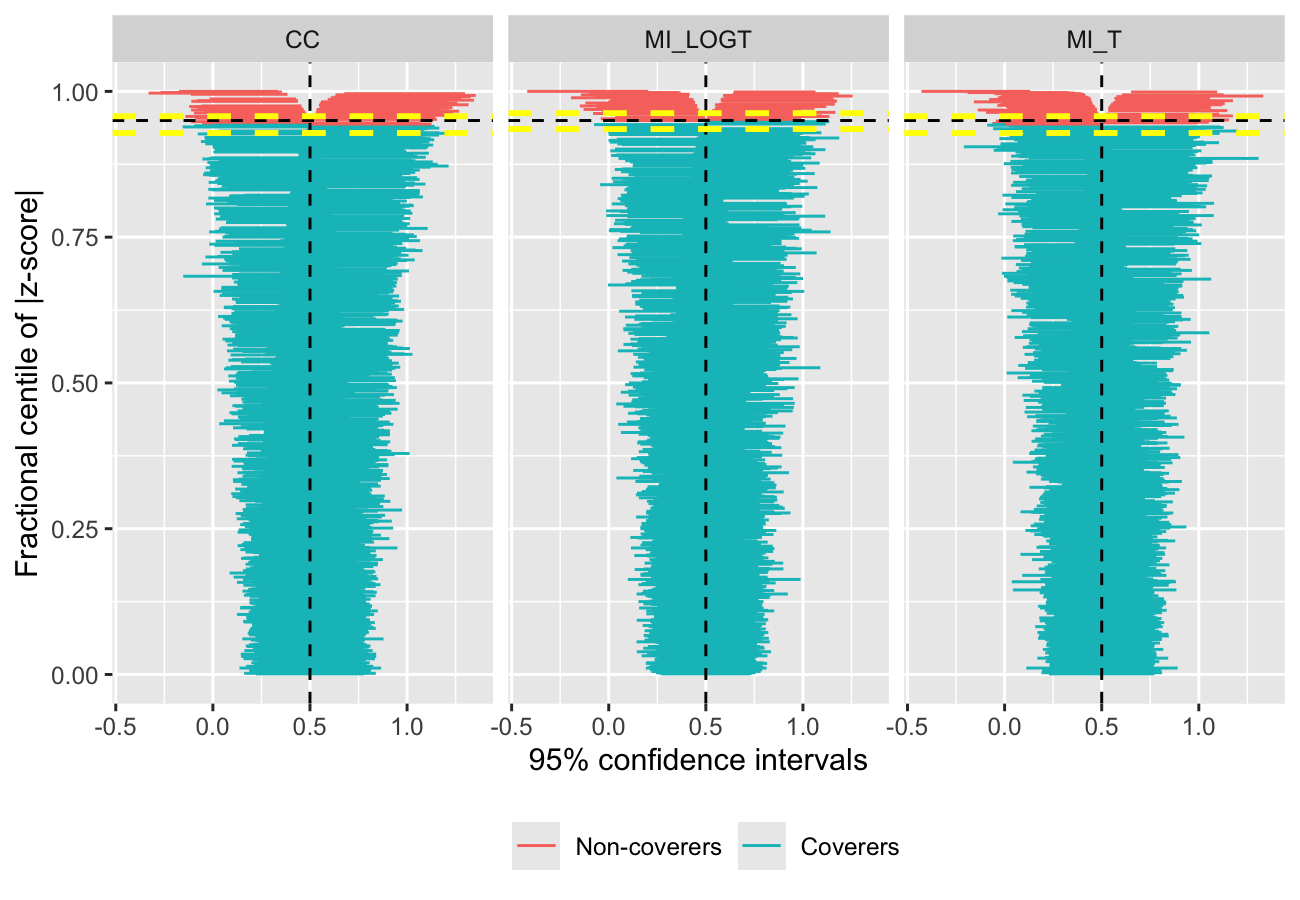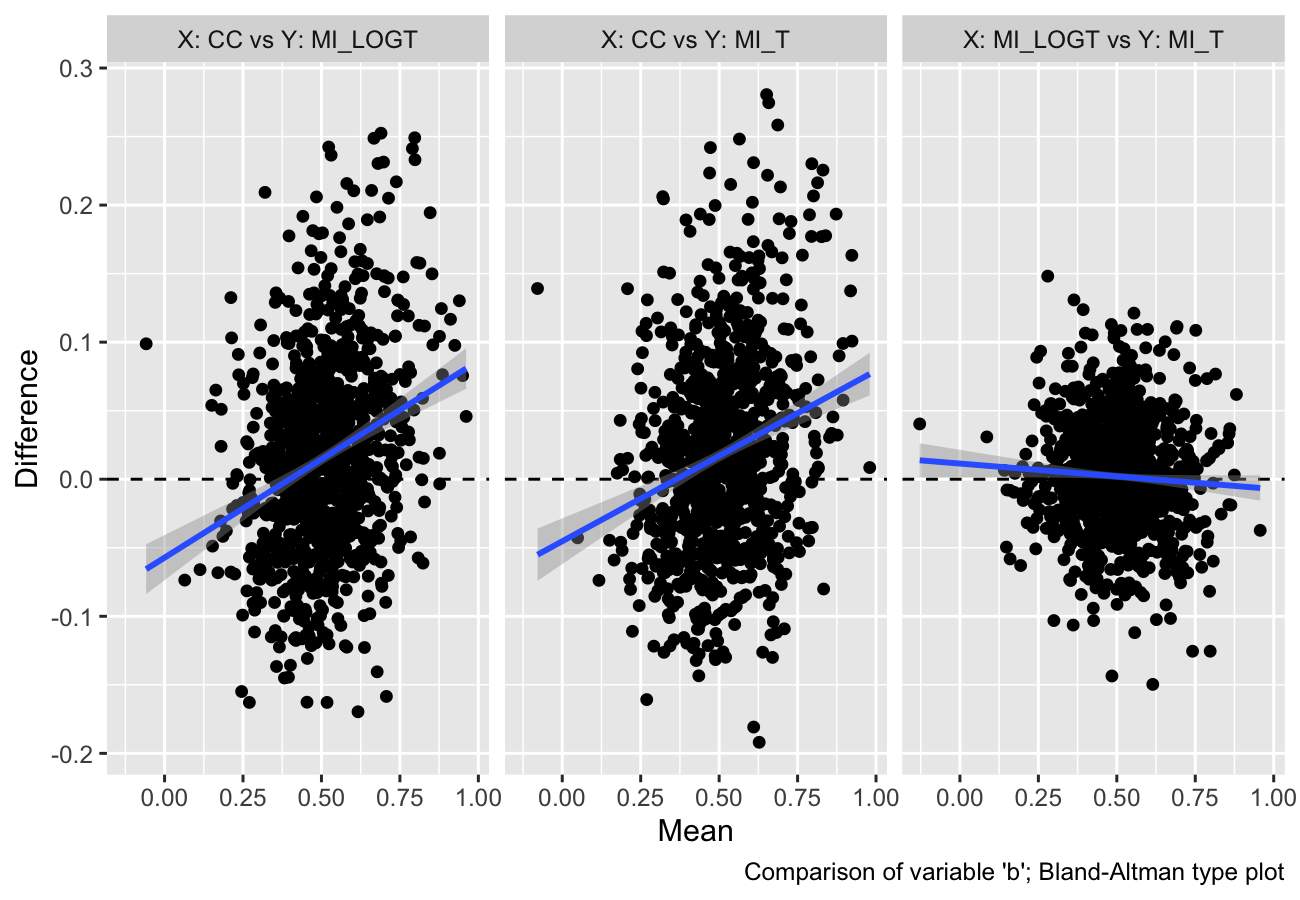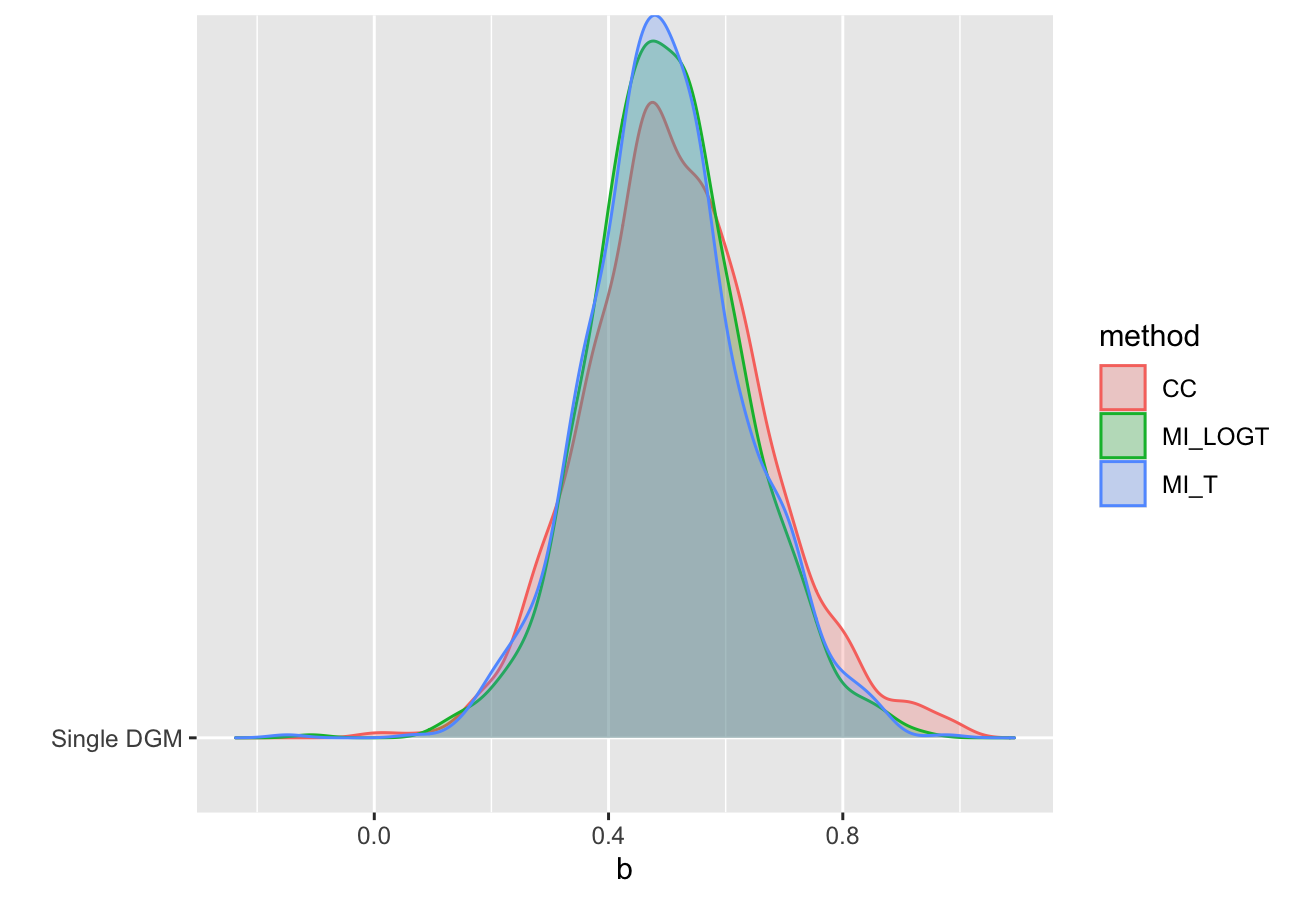# Analysis of Simulation Studies Including Monte Carlo Error

Summarise results from simulation studies and compute Monte Carlo standard errors of commonly used summary statistics. This package is modelled on the 'simsum' user-written command in 'Stata' (White I.R., 2010 < https://www.stata-journal.com/article.html?article=st0200>), further extending it with additional functionality.

`rsimsum` is an R package that can compute summary statistics from simulation studies. `rsimsum` is modelled upon a similar package available in Stata, the user-written command `simsum` (White I.R., 2010).

The aim of `rsimsum` is to help to report simulation studies, including understanding the role of chance in results of simulation studies: Monte Carlo standard errors and confidence intervals based on them are computed and presented to the user by default. `rsimsum` can compute a wide variety of summary statistics: bias, empirical and model-based standard errors, relative precision, relative error in model standard error, mean squared error, coverage, bias. Further details on each summary statistic are presented elsewhere (White I.R., 2010; Morris et al, 2019).

The main function of `rsimsum` is called `simsum` and can handle simulation studies with a single estimand of interest at a time. Missing values are excluded by default, and it is possible to define boundary values to drop estimated values or standard errors exceeding such limits. It is possible to define a variable representing methods compared with the simulation study, and it is possible to define by factors, that is, factors that vary between the different simulated scenarios (data-generating mechanisms, DGMs). However, methods and DGMs are not strictly required: in that case, a simulation study with a single scenario and a single method is assumed. Finally, `rsimsum` provides a function named `multisimsum` that allows summarising simulation studies with multiple estimands as well.

An important step of reporting a simulation study consists in visualising the results; therefore, `rsimsum` exploits the R package `ggplot2` to produce a portfolio of opinionated data visualisations for quick exploration of results, inferring colours and facetting by data-generating mechanisms. `rsimsum` includes methods to produce (1) plots of summary statistics with confidence intervals based on Monte Carlo standard errors (forest plots, lolly plots), (2) zipper plots to graphically visualise coverage by directly plotting confidence intervals, (3) plots for method-wise comparisons of estimates and standard errors (scatter plots, Bland-Altman plots, ridgeline plots), and (4) heat plots. The latter is a visualisation type that has not been traditionally used to present results of simulation studies, and consists in a mosaic plot where the factor on the x-axis is the methods compared with the current simulation study and the factor on the y-axis is the data-generating factors. Each tile of the mosaic plot is coloured according to the value of the summary statistic of interest, with a red colour representing values above the target value and a blue colour representing values below the target.

## Installation

You can install `rsimsum` from CRAN:

Alternatively, it is possible to install the development version from GitHub via:

## Example

This is a basic example using data from a simulation study on missing data (type `help("MIsim", package = "rsimsum")` in the R console for more information):

We set `x = TRUE` as it will be required for some plot types.

Summarising the results:

## Vignettes

`rsimsum` comes with 4 vignettes. In particular, check out the introductory one:

## Visualising results

As of version `0.2.0`, `rsimsum` can produce a variety of plots: among others, lolly plots, forest plots, zipper plots, etc.:With `rsimsum` `0.5.0` the plotting functionality has been completely rewritten, and new plot types have been implemented:

• Scatter plots for method-wise comparisons, including Bland-Altman type plots;• Ridgeline plots.The plotting functionality now extend the S3 generic `autoplot`: see `?ggplot2::autoplot` and `?rsimsum::autoplot.simsum` for further details.

More details and information can be found in the vignette dedicated to plotting:

# Citation

If you find `rsimsum` useful, please cite it in your publications:

# References

• White, I.R. 2010. simsum: Analyses of simulation studies including Monte Carlo error. The Stata Journal 10(3): 369-385 <http://www.stata-journal.com/article.html?article=st0200>
• Morris, T.P., White, I.R. and Crowther, M.J. 2019. Using simulation studies to evaluate statistical methods. Statistics in Medicine, <doi:10.1002/sim.8086>
• Gasparini, A. 2018. rsimsum: Summarise results from Monte Carlo simulation studies. Journal of Open Source Software, 3(26):739 <10.21105/joss.00739>

# Warning for RStudio users

If you use RStudio and equations are not displayed properly within the RStudio viewer window, please access the vignette from the CRAN website or directly from the R console with the command:

This is a known issue with RStudio (see #2253).

# rsimsum 0.5.2

### Bug fixes:

• Fixed labelling when facetting for some plot types, now all defaults to `ggplot2::label_both` for 'by' factors (when included).

# rsimsum 0.5.1

### Bug fixes:

• Fixed calculations for "Relative % increase in precision" (thanks to Ian R. White for reporting this).

# rsimsum 0.5.0

### Improvements:

• Implemented `autoplot` method for `multisimsum` and `summary.multisimsum` objects;
• Implemented heat plot types for both `simsum` and `multisimsum` objects;
• All `autoplot` methods pick the value of `true` passed to `simsum`, `multisimsum` when inferring the target value if `stats = (thetamean, thetamedian)` and `target = NULL`. In plain English, the true value of the estimand is picked as target value when plotting the mean (or median) of the estimated value;
• Updated vignettes and references;
• Updated `pkgdown` website, published at https://ellessenne.github.io/rsimsum/;
• Improved code coverage.

### Bug fixes:

• Fixed a bug in `autoplot` caused by premature slicing of `by` arguments, where no `by` arguments were included.

# rsimsum 0.4.2

Implemented `autoplot` method for `simsum` and `summary.simsum` objects; when calling `autoplot` on `summary.simsum` objects, confidence intervals based on Monte Carlo standard errors will be included as well (if sensible).

Supported plot types are:

• forest plot of estimated summary statistics;
• lolly plot of summary statistics;
• zip plot for coverage probability;
• scatter plot of methods-wise comparison (e.g. X vs Y) of point estimates and standard errors, per replication;
• same as the above, but implemented as a Bland-Altman type plot;
• ridgeline plot of estimates, standard errors to compare the distribution of estimates, standard errors by method.

Several options to customise the behaviour of `autoplot`, see `?autoplot.simsum` and `?autoplot.summary.simsum` for further details.

# rsimsum 0.4.1

Fixed a bug in `dropbig` and related internal function that was returning standardised values instead of actual observed values.

# rsimsum 0.4.0

`rsimsum` 0.4.0 is a large refactoring of `rsimsum`. There are several improvements and breaking changes, outlined below.

### Improvements

• `rsimsum` is more robust to using factor variables (e.g. as `methodvar` or `by` factor), with ordering that will be preserved if defined in the dataset passed to `simsum` (or `multisimsum`);
• Confidence intervals based on Monte Carlo standard errors can be now computed using quantiles from a t distribution; see `help(summary.simsum)` for more details;
• Added comparison with results from Stata's `simsum` for testing purposes - differences are negligible, and there are some calculations in `simsum` that are wrong (already reported). Most differences can be attributed to calculations (and conversions, for comparison) on different scales.

### Breaking changes

• The syntax of `simsum` and `multisimsum` has been slightly changed, with some arguments being removed and others being moved to a `control` list with several tuning parameters. Please check the updated examples for more details;
• `dropbig` is no longer an S3 method for `simsum` and `multisimsum` objects. Now, `dropbig` is an exported function that can be used to identify rows of the input `data.frame` that would be dropped by `simsum` (or `multisimsum`);
• Point estimates and standard errors dropped by `simsum` (or `multisimsum`) when `dropbig = TRUE)` are no longer included in the returned object; therefore, the S3 method `miss` has been removed;
• `get_data` is no longer an S3 method, but still requires an object of class `simsum`, `summary.simsum`, `multisimsum`, or `summary.multisimsum` to be passed as input;
• All plotting methods have been removed in preparation of a complete overhaul planned for `rsimsum` 0.5.0.

# rsimsum 0.3.5

### Breaking changes

• The `zip` method has been renamed to `zipper()` to avoid name collision with `utils::zip()`.

# rsimsum 0.3.4

• Added ability to define custom confidence interval limits for calculating coverage via the `ci.limits` argument (#6, @MvanSmeden). This functionality is to be considered experimental, hence feedback would be much appreciated;
• Updated Simulating a simulation study vignette and therefore the `relhaz` dataset bundled with `rsimsum`.

# rsimsum 0.3.3

`rsimsum` 0.3.3 focuses on improving the documentation of the package.

Improvements:

• Improved printing of confidence intervals for summary statistics based on Monte Carlo standard errors;
• Added a `description` argument to each `get_data` method, to append a column with a description of each summary statistics exported; defaults to `FALSE`;
• Improved documentation and introductory vignette to clarify several points (#3, @lebebr01);
• Improved plotting vignette to document how to customise plots (#4, @lebebr01).

New:

• Added CITATION file with references to paper in JOSS.

# rsimsum 0.3.2

`rsimsum` 0.3.2 is a small maintenance release:

• Merged pull request #1 from @mllg adapting to new version of the `checkmate` package;
• Fixed a bug where automatic labels in `bar()` and `forest()` were not selected properly.

# rsimsum 0.3.1

Bug fixes:

• `bar()`, `forest()`, `lolly()`, `heat()` now appropriately pick a discrete X (or Y) axis scale for methods (if defined) when the method variable is numeric;
• `simsum()` and `multisimsum()` coerce `methodvar` variable to string format (if specified and not already string);
• fixed typos for empirical standard errors in documentation here and there.

Updated code of conduct (`CONDUCT.md`) and contributing guidelines (`CONTRIBUTING.md`).

Removed dependency on the `tidyverse` package (thanks Mara Averick).

# rsimsum 0.3.0

Bug fixes:

• `pattern()` now appropriately pick a discrete colour scale for methods (if defined) when the method variable is numeric.

New plots are supported:

• `forest()`, for forest plots;
• `bar()`, for bar plots.

Changes to existing functionality:

• the `par` argument of `lolly.multisimsum` is now not required; if not provided, plots will be faceted by estimand (as well as any other `by` factor);
• updated Visualising results from rsimsum vignette.

Added `CONTRIBUTING.md` and `CONDUCT.md`.

# rsimsum 0.2.0

Internal housekeeping.

Added S3 methods for `simsum` and `multisimsum` objects to visualise results:

• `lolly()`, for lolly plots;
• `zip()`, for zip plots;
• `heat()`, for heat plots;
• `pattern()`, for scatter plots of estimates vs SEs.

Added a new vignette Visualising results from rsimsum to introduce the above-mentioned plots.

Added `x` argument to `simsum` and `multisimsum` to include original dataset as a slot of the returned object.

Added a `miss` function for obtaining basic information on missingness in simulation results. `miss` has methods `print` and `get_data`.

# rsimsum 0.1.0

First submission to CRAN. `rsimsum` can handle:

• simulation studies with a single estimand
• simulation studies with multiple estimands
• simulation studies with multiple methods to compare
• simulation studies with multiple data-generating mechanisms (e.g. 'by' factors)

Summary statistics that can be computed are: bias, empirical standard error, mean squared error, percentage gain in precision relative to a reference method, model-based standard error, coverage, bias-corrected coverage, and power.

Monte Carlo standard errors for each summary statistic can be computed as well.

# Reference manual

install.packages("rsimsum")

0.9.1 by Alessandro Gasparini, 4 months ago

https://ellessenne.github.io/rsimsum/

Report a bug at https://github.com/ellessenne/rsimsum/issues

Browse source code at https://github.com/cran/rsimsum

Authors: Alessandro Gasparini [aut, cre] , Ian R. White [aut]

Documentation:   PDF Manual